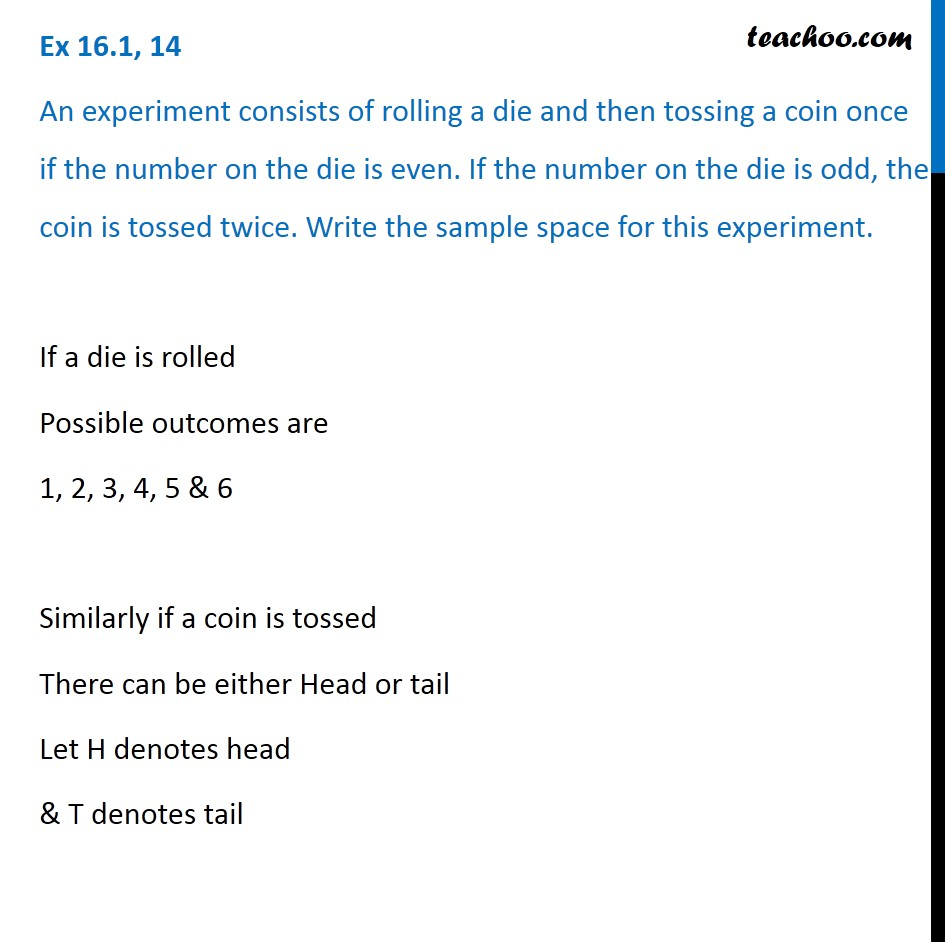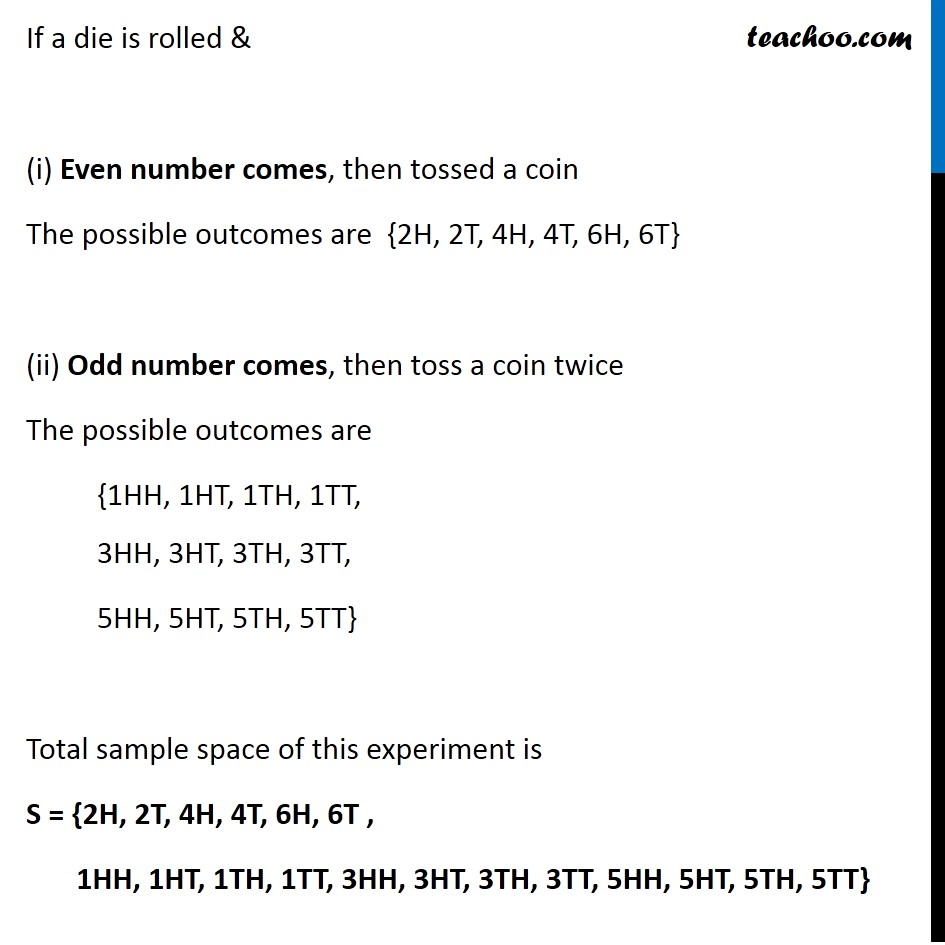Sample Space

Chapter 14 Class 11 Probability
Serial order wiseLearn in your speed, with individual attention - Teachoo Maths 1-on-1 Class

### Transcript

Question 14 An experiment consists of rolling a die and then tossing a coin once if the number on the die is even. If the number on the die is odd, the coin is tossed twice. Write the sample space for this experiment. If a die is rolled Possible outcomes are 1, 2, 3, 4, 5 & 6 Similarly if a coin is tossed There can be either Head or tail Let H denotes head & T denotes tail If a die is rolled & (i) Even number comes, then tossed a coin The possible outcomes are {2H, 2T, 4H, 4T, 6H, 6T} (ii) Odd number comes, then toss a coin twice The possible outcomes are {1HH, 1HT, 1TH, 1TT, 3HH, 3HT, 3TH, 3TT, 5HH, 5HT, 5TH, 5TT} Total sample space of this experiment is S = {2H, 2T, 4H, 4T, 6H, 6T , 1HH, 1HT, 1TH, 1TT, 3HH, 3HT, 3TH, 3TT, 5HH, 5HT, 5TH, 5TT}